Control Theory used in practice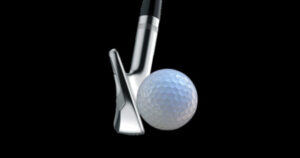##### Physical interpretation of impulse response and its transfer function

I have tried to verify how the function g(t) in the time domain between system inputs and outputs has characteristics depending on the input signal. For this verification, we need an impulse response, so let's start with the main points about an impulse signal. Impulse response is not something we are usually aware of when […]

Feedback control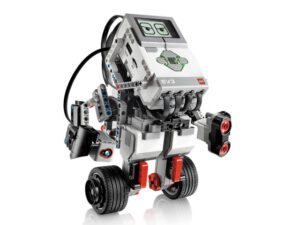##### Tutorial to Feedback Control [ARM used in Practice]

Control Theory Used in Practice What comes to mind when you hear the word "Control"? Although the image may vary from person to person, many people probably imagine programming robots and other devices to operate as envisioned. The kind of control I will be discussing in this site is not so-called sequence control, in which […]

Control Theory used in practice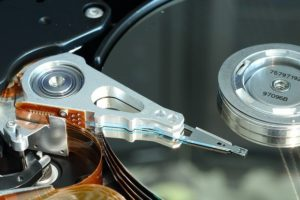##### Fundamentals of Feedback Control using Microcontroller [Advanced]

In "Fundamentals of Feedback Control using Microcontroller [Application]" I explained PID control, which is used in practice based on classical control theory. PID control is easy to use in the field when the model to be controlled is relatively simple, because the gain can be set sensibly. However, when the influence of parts that could […]

Control Theory used in practice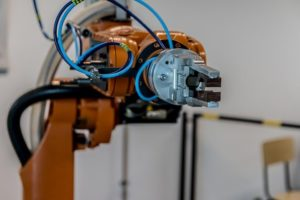##### Fundamentals of Feedback Control using Microcontroller [Application]

In "Fundamentals of Feedback Control using Microcontroller (Analysis)" I have explained the means to improve the characteristics of a control object by modeling it with mathematical formulas and applying feedback, and to improve the tracking of output to a target value by using mathematical formulas as control theory. From now on, I would like to […]

Control Theory used in practice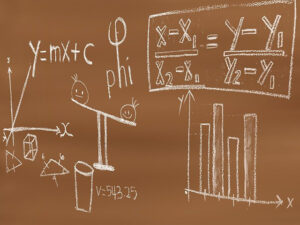##### Fundamentals of Feedback Control using Microcontroller [Analysis]

In "Fundamentals of Feedback Control using Microcontroller [Preparation]" I provide an overview of feedback control and a summary of the process up to the point where the modeled control object is Laplace transformed into complex s-space and a transfer function is created. In this [Analysis] section, I will explain how to read block diagrams and […]

Control Theory used in practice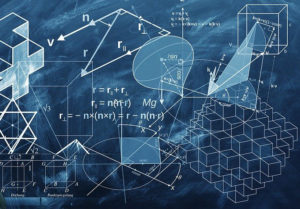##### Fundamentals of Feedback Control using Microcontroller [Preparation]

I have summarized in my own way the outline of control theory, which will expand the world of design and development if it is mastered in order to realize motion control using embedded MCUs. This is not a sequence control in which commands are executed in order as in programming, but a feedback control in […]

Control Theory used in practice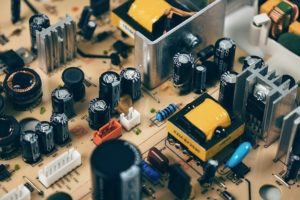##### Actual function of coils and capacitors

The electrical circuits that make up MCU interface are mainly DC circuits, so they are relatively simple, obeying Ohm's law with resistance as the load. However, when it comes to AC circuits, the story is different. This is because the coils and capacitors used in the circuits have a characteristic called impedance, which is the […]